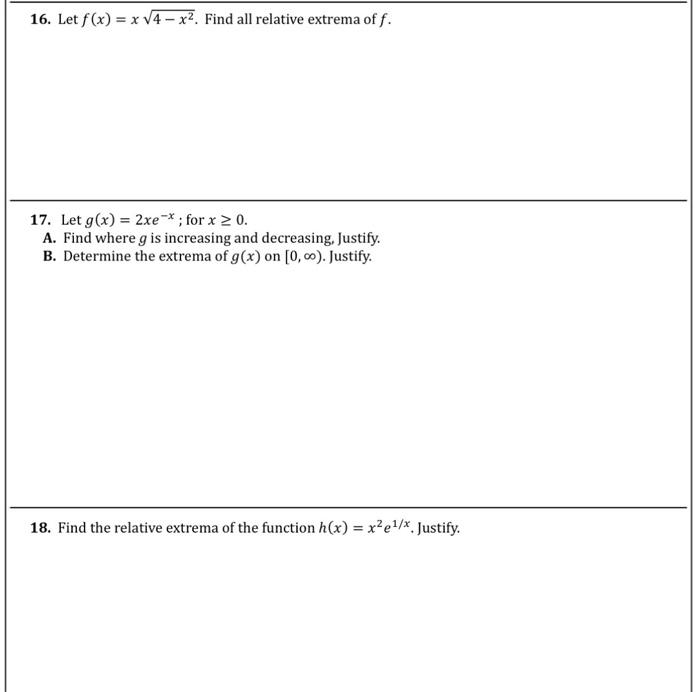### Create an Account

Home / Questions / 16. Let f(x) = x V4 - x2. Find all relative extrema off. 17. Let g(x) = 2xe-*; for x > 0. ...

# 16. Let f(x) = x V4 - x2. Find all relative extrema off. 17. Let g(x) = 2xe-*; for x > 0. A. Find where g is increasing and decreasing, Justify. B. Determine the extrema of g(x) on [0,-). Justify. 18.

16. Let f(x) = x V4 - x2. Find all relative extrema off. 17. Let g(x) = 2xe-*; for x > 0. A. Find where g is increasing and decreasing, Justify. B. Determine the extrema of g(x) on [0,-). Justify. 18. Find the relative extrema of the function h(x) = x?e1/*. Justify.Apr 20 2021 View more View LessSubscribe To Get Solution